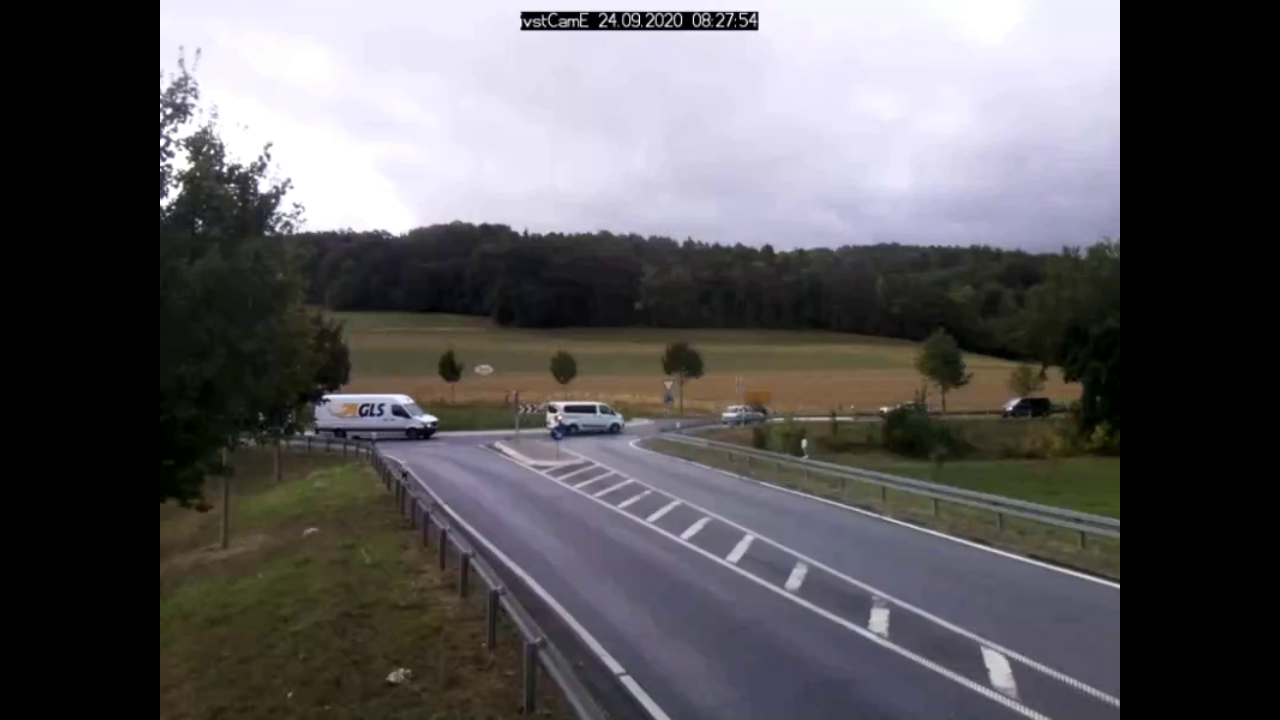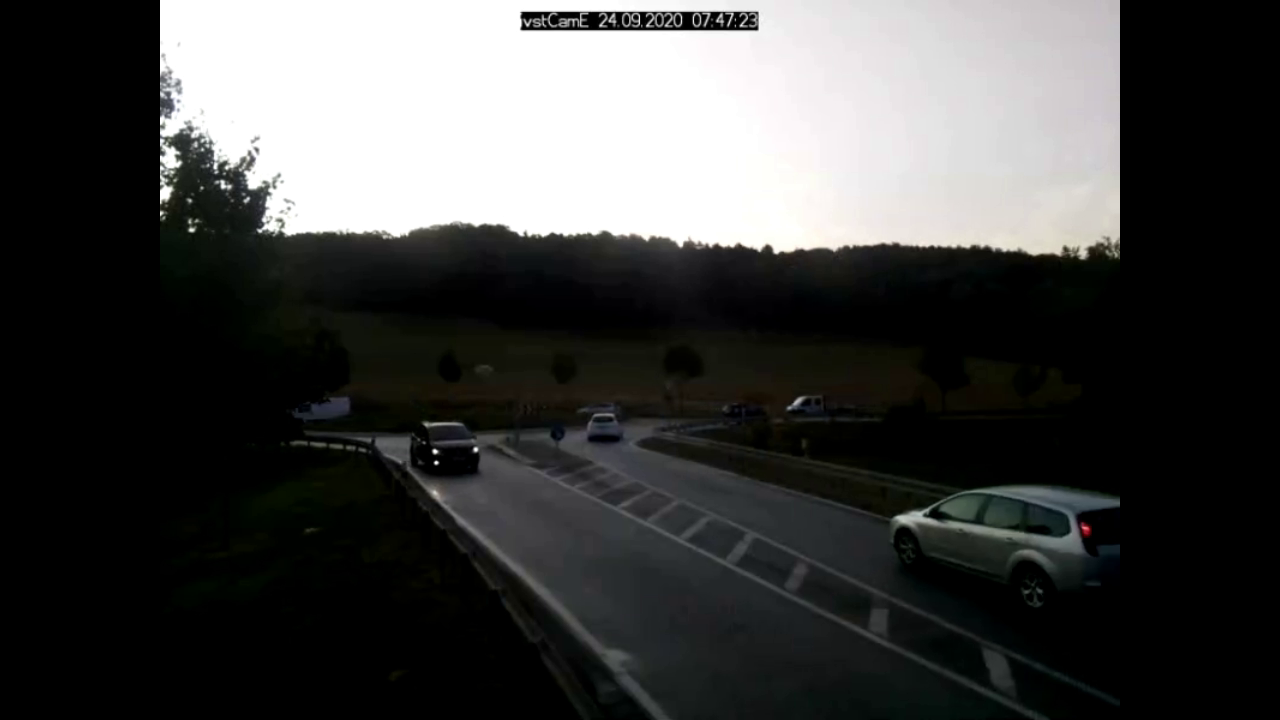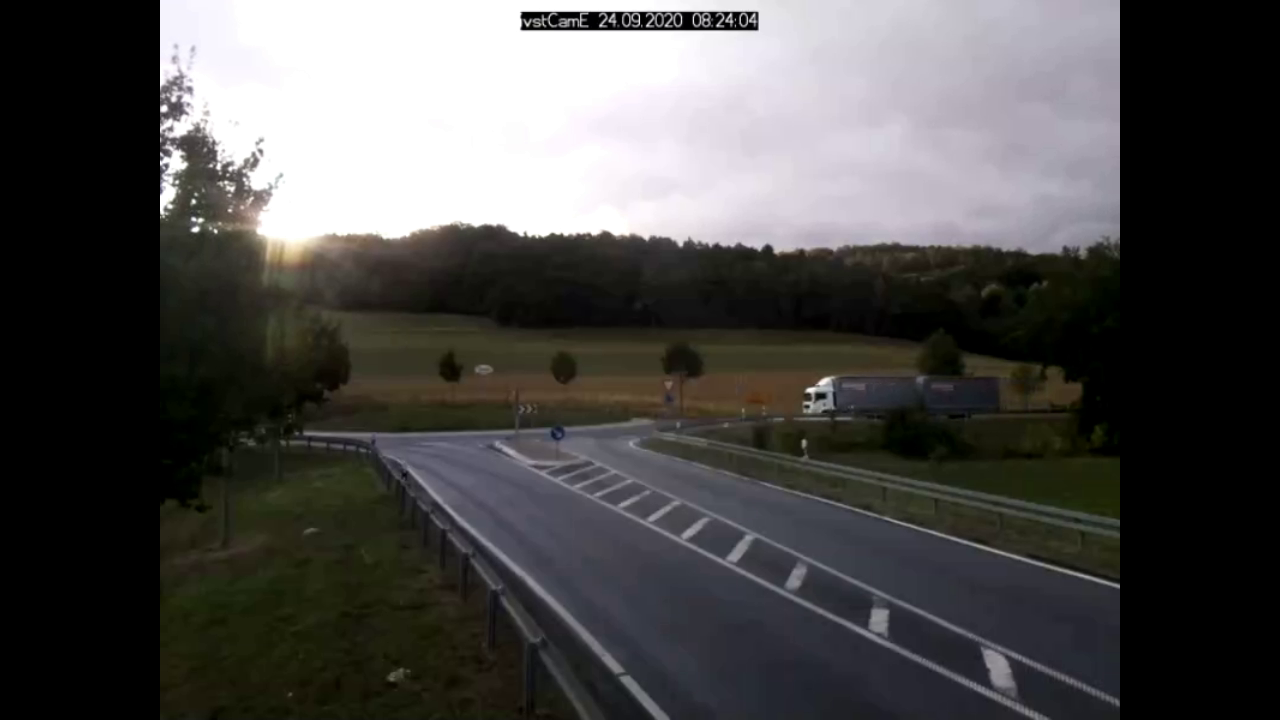# Root Mean Squared Error

Full-Reference Quality Metrics

## Description

The Root Mean Squared Error (RMSE) is the root of the Mean Squared Error (MSE), which is the difference between actual and ideal pixel values. It therefore compares an ideal reference image with an actual image and uses the maximum pixel value for scalation.

## Interpretation

When an image has no pixel differences (no errors), the RMSE equals zero. As image differences increase, its value increases depending on the maximum pixel value.

## Limits

RMSE is simple to calculate but might not align well with the human perception of quality.

Only images showing the same scene should be compared.

## Example

An image from a traffic surveillance camera in Germany is used to show the RMSE results.

Reference ImageRMSE of 45.6RMSE of 30.3## Tools and Libraries

### Python

In Python the package sewar contains multiple image quality metrics. One of them is the RMSE.

Install package:

```pip install sewar
```

Calculate RMSE:

```from sewar.full_ref import rmse
import cv2

score_dark = rmse(img_ref,img_dark)
print("Score of dark image", score_dark)

score_sun = rmse(img_ref,img_sun)
print("Score of dark image", score_sun)
```

### MATLAB

Within the MATLAB Image Processing Toolbox a function to calculate the RMSE doesn’t exists. But because it’s the root of the MSE, which is available in the toolbox, we can calculate it easily.

```ref = imread('Reference_Image.png');

RMSE_dark = sqrt(immse(dark, ref));
fprintf('The RMSE for the dark image is %0.4f\n', RMSE_dark);

RMSE_sun = sqrt(immse(sun, ref));
fprintf('The RMSE for the sun image is %0.4f\n', RMSE_sun);
```

A detailed description can be found at the Mathworks Website. It is also possible to only calculate the RMSE for one channel:

```ref = imread('Reference_Image.png');

RMSE_R = sqrt(immse(dark(:,:,1), ref(:,:,1)));
RMSE_G = sqrt(immse(dark(:,:,2), ref(:,:,2)));
RMSE_B = sqrt(immse(dark(:,:,3), ref(:,:,3)));
fprintf('\nThe RMSE for R-channel %0.4f\n', PSNR_R);
fprintf('The RMSE for G-channel %0.4f\n', PSNR_G);
fprintf('The RMSE for B-channel %0.4f\n', PSNR_B);
```

If access to the MATLAB Image Processing Toolbox is denied, one can program the PSNR by their own:

```num_pixel = size(ref,1)*size(ref,2)*size(ref,3);
MSE = sum((double(ref) - double(dark)).^2,'all') / num_pixel;
RMSE = sqrt(MSE);
fprintf('The RMSE for the dark image is %0.4f\n', RMSE);
```

### C++

OpenCV doesn’t contain a class for calculating the RMSE. But because it’s the root of the MSE, which is a class in OpenCV, we can calculate it easily.

```#include <opencv2/quality/qualitymse.hpp>
#include <iostream>
#include <math.h>

int main()
{
std::string image_path = samples::findFile("Reference_Image.png");
std::string image_path = samples::findFile("Image_Dark.png");